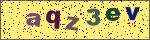# PHP中echo(),print(),print_r(),printf()的区别

echo(), print(),print_r()区别在于

1. echo 可以输出多个变量值,而print只有一个变量，做为一个字符串输出.
2. echo 没有返回值, print有返回值1.
3. print不能输出数组和对象。print_r 可以输出stirng、int、float、array、object等，输出array时会用结构表示，print_r输出成功时返回true； 而且print_r可以通过print_r(\$str,true)来使print_r不输出而返回 print_r处理后的值。 可以理解为：print 是打印字符串print_r 则是打印复合类型（如数组，对象等）
4. 在PHP中的执行速率从快到慢为：echo(),   print(),   print_r()
```echo是PHP语句, print和print_r是函数,语句没有返回值,函数可以有返回值(即便没有用)
print（）    只能打印出简单类型变量的值(如int,string)
print_r（） 可以打印出复杂类型变量的值(如数组,对象)
echo     输出一个或者多个字符串```

```void echo ( string arg1 [, string ...] ) //返回值为空
echo "你好"," 朋友";```
```print --输出一个字符串
Description
int print ( string arg )//返回值为整形
print "你好朋友";```

```\$name=print "nihao \n";
\$str = 'test print value is \$name .';
eval_r("\\$print=\"\$str\";");
echo \$print; ```

print_r -- 打印关于变量的易于理解的信息。

```bool print_r ( mixed expression [, bool return] ) //返回值是布尔型的,参数是mix类型的,可以是字符串,整形,数组,对象类print_r() 显示关于一个变量的易于理解的信息。如果给出的是 string、integer 或 float，将打印变量值本身。如果给出的是 array，将会按照一定格式显示键和元素。object 与数组类似。

print_r() 将把数组的指针移到最后边。```

```print_r(str);
print_r(int);
print_r(array);
print_r(obj);

echo()

print()

```\$a=print('hi');
echo \$a;

hi 1
//1是\$a的值。
```

die();  和exit()区别:

mysql_connect("locahost","root","root") or die("链接服务器失败！");

```printf();    //f指format格式化
printf("参数1",参数2)：参数1=按什么格式输出；参数2=输出的变量。

(%s:按字符串;%d: 按整型;%b:按二进制；%x:按16进制；%X：按16进制大写输出；%o:按八进制; %f:按浮点型)

【-】是可选的，添加它则表明数据应该左对齐。而不是默认的右对齐，如上例加一个-则为：printf ("\$%'a-10.2f" , 43.2); //\$43.20aaaaa
whidth 表示在这里为将被替换的变量留下多少空间（按字符计算）。如上例的10(包括小数点).
precision则必须是一个小数点开始，表示小数位后面要显示的位数。

printf ("\$.2f" , 43.2); //\$43.20
\$表示填充的字符
0表示位数不够在不影响原值的情况下补0
1表示输出的总宽度
2表示小数位数，有四舍五入
%f 是表示显示为一个浮点数

%% 印出百分比符号，不转换。
%b 整数转成二进位。
%c 整数转成对应的 ASCII 字符。 如：printf ("\$%c" , 65); // 输出：A
%d 整数转成十进位。 如：printf ("\$%d" , 65.53); // 输出：65
%f 倍精确度数字转成浮点数。
%o 整数转成八进位。
%s 整数转成字符串。
%x 整数转成小写十六进位。
%X 整数转成大写十六进位

printf ("the total is \$%2\$.2f and subtotal: %1\$.2f" , 65.55,37.2); //the total is \$37.20 and subtotal: 65.55

#### 发表评论